Home | | Engineering Chemistry II | Electro Chemistry

# Electro Chemistry

1 Introduction 1.1 Types of cells 2 Electrochemical Cells 2.1 Reversible and Irreversible cells 3 Electrode Potential 3.1 Reduction Potential 3.2 Single electrode Potential 3.3 Standard electrode Potential 3.4 Measurement of Standard electrode Potential 4 EMF of Cell 4.1 Measurement of EMF of cell 4.2 Applications of EMF measurement 5 Electrochemical Series 5.1 Significance of EMF series 6 Nernst Equation 6.1 Nernst equation for reversible cell 6.2 Problems based on Nernst equation

ELECTRO CHEMISTRY

1 Introduction

1.1 Types of cells

2 Electrochemical Cells

2.1 Reversible and Irreversible cells

3 Electrode Potential

3.1 Reduction Potential

3.2 Single electrode Potential

3.3 Standard electrode Potential

3.4 Measurement of Standard electrode Potential

4 EMF of Cell

4.1 Measurement of EMF of cell

4.2 Applications of EMF measurement

5 Electrochemical Series

5.1 Significance of EMF series

6 Nernst Equation

6.1 Nernst equation for reversible cell

6.2 Problems based on Nernst equation

1 INTRODUCTION

Electrochemistry is the branch of science which deals with the relationship between chemical reaction and electricity. Electrochemistry is the study of the process involved in theinterconversion of electrical energy to chemical energy and vice – versa

Electric current is a flow of electrons generated by a cell or a battery when the circuit is completed. A substance which allows electric current to pass through it ,is called a conductor, e.g. metals, graphite, fused metallic salts while non – conductor is a substance which does not conduct the electric current e.g. plastics, wood, non – metals ect,

IMPORTANT TERMS INVOLVED IN ELECTROCHEMISTRY

Cell: Cell is an assembly of two electrodes and an electrolyte.Generally, it consists of two half cells. Each half cell contain an electrode material in touch with electrolyte.

Current:Current is a flow of electricity through a conductor. It is measured in ampere. Electrode is a material rod/bar/strip which conduct electrons.

Anode: Anode is an electrode at which oxidation occurs

Cathode: Cathode is an electrode at which reduction occur

Electrolyte: Electrolyte is a liquid or solution that conducts electric current. There are three types of electrolytes.

(a) Strong electrolytes: these are the substances which ionize completely at any concentration.

Example: HCL,aqueous solutions of NAOH, NaCl and KCL

(b)Weak electrolytes: Weak electrolytes are the substance which ionize partially in solution.

Example: CH COOH, NH OH and aqueous solution of Na CO

Non electrolyte: Non electrolyte are the substances which do not ionize at any dilutions.

Example: Glucose, Sugar,alcohol, petrol,etc.

Galvanic (or) Voltaic (or) Electrochemical cell

It is a device that produces electrical energy at the expense of chemical energy produced in a reaction.

A cell consists of two half cells or electrodes.

A half-cell or electrode contains a metal rod dipped in an electrolytic solution. Electrolytic                              cell:  It  is  a  cell  in  which  electrical  energy  brings

Electrochemical cell: It is a device that produces electrical energy at the expense of chemical energy produced in a reaction.

Differences between electrolytic cell and electrochemical cell.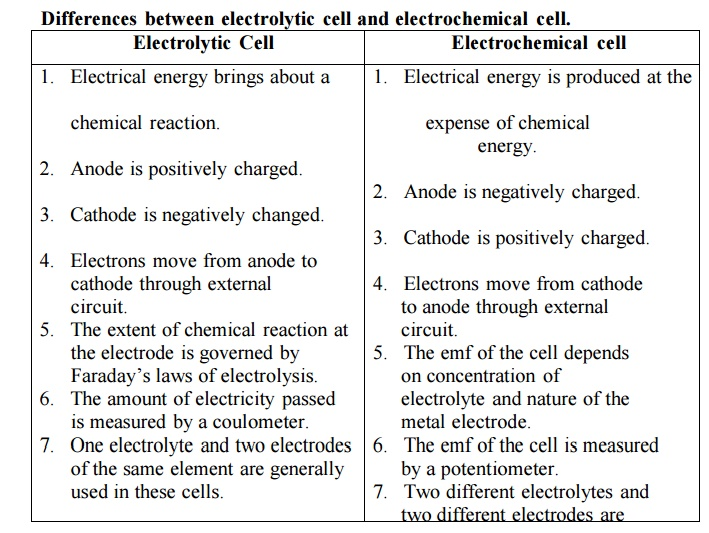Electrolytic Cell

1.       Electrical energy brings about a chemical reaction.

2.       Anode is positively charged.

3.       Cathode is negatively changed.

4.       Electrons move from anode to cathode through external circuit.

5.       The extent of chemical reaction at the electrode is governed by Faraday‟s laws of electrolysis.

6.       The amount of electricity passed is measured by a coulometer.

7.       One electrolyte and two electrodes of the same element are generally used in these cells.

Electrochemical cell

1.       Electrical energy is produced at the expense of chemical energy.

2.       Anode is negatively charged.

3.       Cathode is positively charged.

4.       Electrons move from cathode to anode through external circuit.

5.       The emf of the cell depends on concentration of electrolyte and nature of the metal electrode.

6.       The emf of the cell is measured by a potentiometer.

7.       Two different electrolytes and two different electrodes are

1.1 TYPES OF CELL

Electrolytic cell

It is a device in which chemical reaction proceed at the expanse of electrical energy.

Ex. Electro plating and electrolysis Electrolysis of NaCl

The cell is constituted by dipping two platinum electrode in an appropriate electrolyte ( NaCl in water ) . The electrodes are connected to the two terminals of a battery. The electrode connected to positive terminal acts as anode (attracts anions) and the other electrode connected to the negative terminal acts as cathode (attracts cations). Chlorine is liberated at anode and hydrogen is liberated at cathode

Cell reaction:

At anode: 2 Cl   --- >  Cl2 ↑ + 2e

At cathode: (i) Na + + H2O  --- >  NaOH + H+

(ii) 2 H+  + 2e -- -- > H2

Net reaction:  2NaCl  + 2H2O ▬▬► 2NaOH + H2     + Cl2ECTROCHEMICAL CELL:

consists of two half-cells joined by a salt bridge or some other path (porous membrane)that allows ions to pass between the two sides in order to maintain electro neutrality.

oxidation occurs at one half cell  while reduction takes place at the other half cell.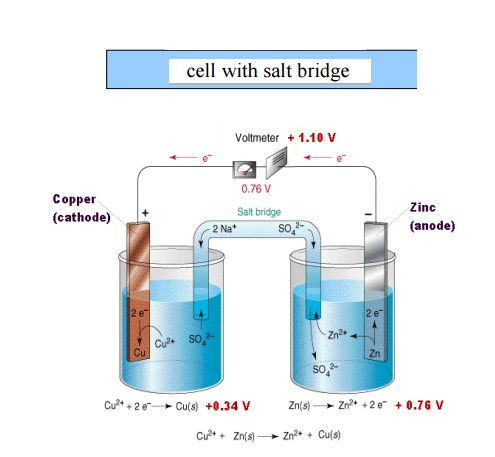Note:Liquid junction potential is higher when porous membrane is used for joining two half cells while it is minimum in a cell with a salt bridge.

Liquid junction potential occurs when two solutions of different concentrations/compositions are in contact with each other through porous membrane. It is due to formation of an electrical double layer of positive and negative charges at the junction of the two solutions as the ions diffuse through membranes.

Salt bridge: Contains a solution of a salt (KCl or KNO 3 or NH4NO3) that literally serves as a bridge to complete the electric circuit, maintain electro neutrality of electrolyte

and minimize the liquid junction potentia. For precise measurements of potential, a salt bridge is used.

Fundamental components of electrochemical cell

1.Anode: oxidation half-reaction takes place ;Oxidation of metal releases metal ions into the solution of oxidation half-   cell, leaving behind the electron at the surface of the metal electrode.

2.Cathode: reduction half-reaction occurs;Movement of metal ions from the solution to the electrode to gain es in reduction half cell leads to accumulation of positive charge at the surface of the electrode.

Electrolyte:Internal conducting environment that allows ions to migrate between both half cells so as to preserve electro neutrality

External circuit : Two half-cells are joined together by wire through which electrons flow.

Salt bridge/porous membrane: Serves as a bridge to complete the

electric circuit and maintain electro neutrality in the electrolyte.

2 Electrochemical Cell or Galvanic cell

It is a device in which a redox reaction is used to derive electrical energy. During the working of the cell the stored chemical energy decreases and this decrease is gained as electrical energy.

In the electrochemical cell the electrode at which oxidation occurs is called anode (− ve) and the electrode at which reduction occurs is called cathode (+ ve).

Example:  Zn acts as anode and Cu acts as cathode in Daniel cellIt consists of zinc electrode dipped in 1M zinc sulphate solution and a copper electrode dipped in 1M copper sulphate solution. Each electrode acts as a half cell connected by a salt bridge through a voltmeter. The two solutions can seep through the salt bridge without mixing.Representation of a galvanic cell

Galvanic cell consists of two electrodes, anode and cathode

The anode is written on the left hand side while the cathode is written on right side.

The anode is written with the metal first and then the electrolyte .The two are separated by a vertical line or semicolon

Ex:   Zn / Zn 2+ (or) Zn / ZnSO4 (or) Zn; Zn 2+

(iv) The cathode is written with electrolyte first and then the metal both are separated by vertical line or semicolon(v) The two half cells are connected by a salt bridge which is indicated by two parallel lines.Salt bridge: It consists of a U tube filled with a saturated solution of KCl or (NH4)2NO3 in agar-agar gel. It connects the two half cells and performs the following

functions

It eliminates the liquid junction potential.

It provides path for the flow of electrons between two half cells.

Completes the circuit.

Maintains electrical neutrality in the two compartments by migration of ions through the porous material thus ensures the chemical reactions proceed without hindrance

Prevents mixing of the electrode solutions

2.1 Reversible cells

A cell works reversibly in the thermodynamic conditions. Ex. Daniel cell, Secondary batteries, Rechargeable batteries.

The cell is reversible if it satisfies all the following conditions:

If applied emf is equal to derived emf then the net reaction is zero

If applied emf is infinitesimally smaller than the derived emf then the cell should act as electrochemical cell (forward reaction)

If applied emf is infinitesimally greater than the derived emf then the cell should act as electrolytic cell (reverse reaction)

Irreversible cells

Cells which do not obey the (above) conditions of thermodynamic reversibility are called irreversible cells. If one of the products escapes from the cell then that cell cannot be made reversible by applying an external current.

Ex. Zinc-Silver cell, Primary cells

Zn –Ag Cell

Zn / H2SO4 (aq) / Ag

Cell reaction:When two electrodes are connected from outside, zinc dissolves liberating hydrogen gas. Since one of the product hydrogen escapes, the cell reaction cannot be reversed when connected to an external EMF. The cell does not obey the conditions of reversibility and is called irreversible cell.

3 Electrode Potential :

3.1 Oxidation potential

When a metal M is dipped in its salt solution, one of the following reactions occurs depending on the metal :

Positive metal ions pass into the(e.g.) When Zn rod is dipped in ZnSO4 solution, Zn goes into solution as Zn2+.

The electrons attach to Zn rod, giving it a negative charge. The negative charge on the rod attracts positive ions from solution. Thus a double layer of ions is formed close to the rod.3.2 REDUCTION POTENTIAL

2.     Positive ions from the solution deposit over the metal.When Cu rod is dipped in CuSO solution, Cu+2 ions from the solution deposit on metal rod. They attract negative ions from solution. Thus a double layer of ions is formed close to the metal rod. This is called Helmholtz double layer.As a result a potential difference is set up between the metal and the solution. The equilibrium value of the potential difference is known as electrode potential.

Factors affecting electrode potential or emf of Cell :

nature of the metal

temperature

concentration of metal ions in the solution

3.3 Single electrode potential (or) electrode potential :

It is a measure of the tendency of the metal electrode to lose or gain electrons, when it is in contact with its own salt solution. It is developed due to the formation of a double layer around the metal rod.

3.4 Standard electrode potential :

It is a measure of the tendency of the metal electrode to lose or gain electrons, when it is in contact with its own salt solution of 1M strength at 25C.

Measurement of single electrode potential :

It is impossible to determine the value of a single electrode potential. But we can always measure the potential difference between two electrodes using a potentiometer, by combining the two electrodes to form a cell. For this purpose we reference electrode. Standard hydrogen electrode is called primary reference electrode. Calomel electrode is called secondary reference electrode.

Standard hydrogen electrode (SHE) (Primary Reference Electrode)

It has a platinum foil connected to platinum wire and sealed in a glass tube. The platinum foil is dipped in 1M HCl. Hydrogen gas 1 atm pressure is passed through the side arm of glass tube as shown in the figure. The standard electrode potential of SHE is taken as zero. The electrode is represented,The electrode reaction isLimitations (or) drawbacks of SHE:

H2 gas reduces many ions like Ag+ and affects compounds of Hg, Ag etc.

It is difficult to get pure H2.

The pressure of H2 is to be kept 1 atm all the time.

It is difficult to set up and transport.

The electrode potential changes with barometric pressure.

6) A large volume of test solution is         required.

It cannot be used in solutions of redox systems.

The solution may poison platinum surface. So we use a secondary reference electrode.

Calomel Electrode (Secondary reference electrode):

It consists of a glass tube containing pure mercury at the bottom. A paste of mercurous chloride covers the mercury. A solution of potassium chloride is present over the paste. The bottom of the tube is sealed with a platinum wire. There is a side tube for electrical contact. The electrode is represented as,

Hg | Hg2Cl2(s) | KCl(aq)

The electrode reaction is,At 25oC,

E = E – 0.0591 log (Cl-)

For saturated KCl, E         = +0.242 volt.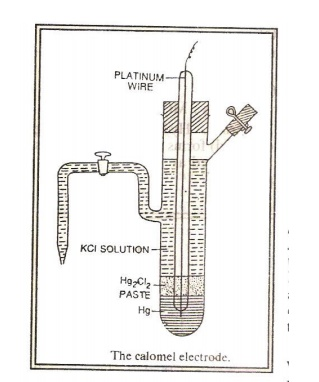Measurement of single electrode potential using a reference electrode (saturated calomel electrode):

The given electrode, say zinc electrode, is coupled with saturated calomel electrode as in the figure. Since the reduction potential of zinc electrode less than that of calomel electrode, zinc acts as anode and calomel as cathode. The cell reaction will be

Zn/ ZnSO4 (1 M) // KCl (satd )/ Hg2Cl2/HgThe emf of the cell is measured using a potentiometer.  The value of Ecell = 1.002 volt.

Now, Ecell = EOright – E0left

= EOcal – EOZn

1.002 = 0.242 – E0Zn

EZn  = 0.242-1.002

EZn  = - 0.76 volt.

Advantages of Reference Electrode (Calomel Electrode):

Easy to set up.

Easily transportable

Long shelf life

Reproducibility of emf

Low temperature coefficient

Electrode can be used in a variety of solutions.

Eo value is accurately known.

Ion sensitive electrode :

Ion sensitive electrodes have the ability to respond only to a specific ion and develop a potential ignoring other ions in the solution.

The types (classification) of ion-sensitive electrode :

Glass membrane electrodes

Solid state electrode

Pungor or precipitate electrodes

Liquid – liquid electrode

Glass Electrode (or) Measurement of pH using glass electrode :

Glass electrode contains a thin-walled glass bulb. The glass has low melting point and high electrical conductivity. 0.1M HCl is present in the bulb. A platinum wire is inserted in the acid.

When      the   glass        membrane                  separates  two  solutions      differing         in  pH, exchange of H+ ions takes place between the solutions. As a result a potential is developed across the membrane. The potential EG is given by,

EG = EG + 0.0591 pH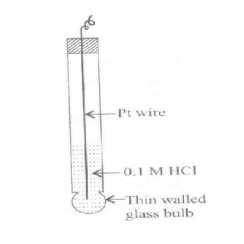Measurement of pH :

The glass electrode is dipped in the given solution. This system is connected to saturated calomel electrode as in the figure. The emf of the resulting cell is measured using a potentiometer.

From the emf, the pH of the solution is calculated as

below: Ecell    = Eright – Eleft

Ecell= Ecal – Eglass

Ecell = 0.242 – (EG° + 0.0591v pH)

Ecell = 0.242 - EG° - 0.0591 pH

PH =Ecell – 0.2422+E0Glass/0.0592i)It is easily constructed and used

ii)Results are accurate iii)Electrode is not easilpoisoned iv) Equilibrium is quickly attained

v) It can be used  in strong  oxidizing  solutions,  coloured  solutions  and

in presence of metal ions

vi) Using special glass electrode, pH can be measured from 0 to 12.

vii) It is used in chemical, industrial, biological and agricultural laboratories.

i)      Glass has high  resistance.     So       special     electronic       potentiometer

must  be used.

It cannot be used in highly alkaline solutions, in pure ethanol or in acetic acid. If the solution pH is more than 12, glass membrane is affected by cations.

Applications of Ion-sensitive electrode :

To determine ions like H+, K+, Li+, etc.

To determine hardness of water (Ca+2 and Mg+2 ions)

To determine concentration of F-, NO3-, CN- etc.

To determine concentration of a gas using gas-sensing electrodes.

v) To determine pH of a solution using H+ ion sensitive electrode.

4 EMF of an electrochemical cell

Difference of potential which causes the flow of current from the electrode of higher potential to one of lower potential

It is the algebraic sum of the single electrode potentials provided the proper sign are given according to actual reaction taking place on the

Nernst eqn for electrochemical cell

ECell=E right-Eleft,       or Ecell =E cathode -Eanode

E° cell+ 0.0592Vlog[reactant]/[p roduc)

4.1Instrument:

emf of the cell can be measured by potentiometer-using Poggendorff’s compensation principle(wheatstone's bridge).

Voltmeter cannot be used to determine accurate value of emf because during measurement a part of cell current is drawn which causes change in the emf.

Factors affecting the emf of a cell

1.Nature of the electrolytes and electrodes. 2.Concentration and composition of the electrolytes. 3.pH and temperature of the solution.

4.2 Applications of EMF Measurements

The valency of an ion can be determined.

Solubility of a sparingly soluble salt can be determined.

Potentiometric titrations can be carried out.

Hydrolysis constant can be determined.

Determination of standard free energy change and equilibrium constant

Determination of pH by using a standard hydrogen electrode.

5 ELECTROCHEMICAL SERIES (e.m.f series)

A series in which elements are arranged in the ascending (increasing ) order Of their standard reduction potential is called emf series.

5.1 Significance of EMF seriesApplication / Significance of electrochemical series

(i) Relative ease of oxidation or reduction

• The metals which lie above hydrogen in the series undergo spontaneous oxidation and the metals which lie below SHE undergo reduction spontaneously ( ie. Acts as Anodes and Cathodes respectively)

• The metals which lie above hydrogen are good reducing agents and which lies below hydrogen will act as good oxidizing agents

(ii) Replacement tendency

The metal lying above in emf series displaces the metal lying below it from an electrolyte of the later.

Example 1: Ni spatula cannot be used to stir copper sulphate solution due to the following reactionExample 2: when zinc is dipped in copper sulphate solution copper gets deposited (displaced)(iii) Liberation of Hydrogen

• The metal with negative reduction potential will displace H2 from an acid solutionHence acids cannot be stored in galvanized steel containers.

For exactly the same reason galvanized steels are not used to store food stuffs containing vinegar. (Vinegar is used as food preservative- vinegar is acetic acid)

(iv) Calculation of equilibrium constant (Keq)(v) Calculation of Standard emf of the cell

E cell  =  E cathode     −  E anode

( if both reduction potentials are considered)

E cell  =  E cathode     +  E anode

( if oxidation potential of anode and the reduction potential of cathode are considered)

Corrosion

The metals higher in the series are anodic and are more prone to corrosion.

The metals lower in the series are noble metals (cathodic) and they are less prone to corrosion.

Predicting the spontaneity of cell reaction

Spontaneity of the redox reaction can be predicted from the emf value of complete cell reaction.

If the value of Ecell    is positive, the reaction is feasible. as ∆G will be negative

( i.e. it is an electrochemical cell)

If the value of Ecell    is negative, the reaction is not feasible. as ∆G will be positive

( i.e. it is an electrolytic cell)

6 NERNST EQUATION

If an emf of a reversibile cell is E volts and the cell reaction that takes place is accocated with the passage of an faradays or nF coulombs, the electrical work done by the system is given by the product of the emf and total charge.

Electrical work done by the system = nFE, where E is the reversibile emf of the cell and n is the no.of electrons involved in the cell reaction.

ΔThe work done on the system at constant temperature is the increase in the free energy ΔG of the system.

The free energy change of a reaction depends on the activities of the reactants and the products. Therfore, in the case of cell reactions of a reversibile, the emf of the cell will also depend on the activities of the reactant and products.

Applications of Nernst Equation :

I)To calculate electrode potential of unknown metal. ii)To predict corrosion of metals.

iii) To set up electrochemical series.

6.1Nernst equation for a reversible cell :

Let us consider the reaction in a reversible cell :The free energy change ΔG of this equilibrium  is related to the equilibrium constant K by the Van‟t Hoff isotherm.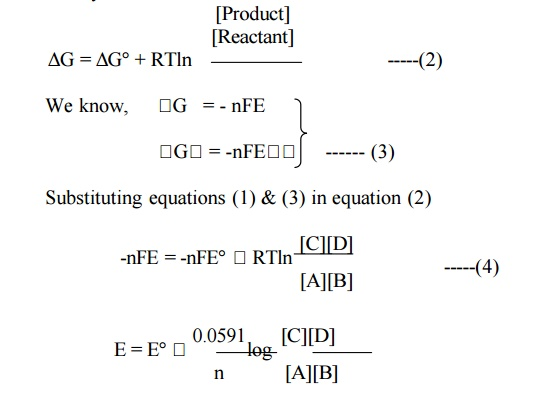This is Nernst equation for a reversible cell.

6.2 PROBLEM BASED ON NERNST EQUATION

Calculate the reduction potential of lead electrode in contact with a solution of 0.015M Pb2+ ions.( E0 = -0.13 volt)

GIVEN

Std Oxidation potential is given as Pb2+ + 2 e- → Pb; E0 = -0.13v

Concentration of  Pb2+ = 0.015M

Solution

The Nernst equation for reduction potential isOxidation potential of Pb = -0.1839V

Study Material, Lecturing Notes, Assignment, Reference, Wiki description explanation, brief detail
Chemistry : Electro Chemistry and Corrosion : Electro Chemistry |